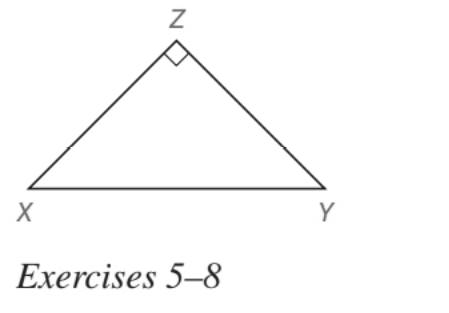Chapter 5.5, Problem 5EElementary Geometry For College St...

7th Edition
Alexander + 2 others
ISBN: 9781337614085

Solutions

Chapter
SectionElementary Geometry For College St...

7th Edition
Alexander + 2 others
ISBN: 9781337614085
Textbook Problem

In Exercises 5 to 22, find the missing lengths. Give your answers in both simplest radical form and as approximations correct two decimal places. Given: Right ∆ X Y Z with m ∠ X = 45 ° and X Z = 8 Find Y Z and X YTo determine

To find:

YZ and XY for the right XYZ with mX=45° and XZ=8.

Explanation

Approach:

For a right triangle for which the measure of the interior angles 45°, 45°, and 90°, if ‘a’ is the length of measure of one of the leg; opposite to the angle 45°, then the length of the other two sides is given by

Length of the other leg =a

Length of the hypotenuse =a2

In general

Length of the legs are equal.

Length of the hypotenuse =2× (Length of one of the legs)

Calculation:

Given,

The right XYZ with mX=45° and XZ=8.

The given triangle should be a 45°-45°-90° triangle since its is given that one of the acute angle mX of the right triangle is given as 45°

Thus,

mX=45°

Thus, the triangle become

Still sussing out bartleby?

Check out a sample textbook solution.

See a sample solution

The Solution to Your Study Problems

Bartleby provides explanations to thousands of textbook problems written by our experts, many with advanced degrees!

Get Started

Find the derivatives of the functions in Problems 1-34. 15.

Mathematical Applications for the Management, Life, and Social Sciences

Evaluate the integral, if it exists. 0/4(1+tant)3sec2tdt

Single Variable Calculus: Early Transcendentals, Volume I

Elementary Technical Mathematics

Expand each expression in Exercises 122. (2xy)y

Finite Mathematics and Applied Calculus (MindTap Course List)

Domain Find the domain of the function. 69. f(x)=3x4

Precalculus: Mathematics for Calculus (Standalone Book)

y=4x2+1 defines y implicitly as a function of x.

Study Guide for Stewart's Single Variable Calculus: Early Transcendentals, 8th